In [ ]:



# Genotype quality control¶

This tutorial explores typical genotype quality control metrics, including allele frequency distribution, linkage disequilibrium and methods to test for population structure.

## Allele frequency distribution¶

Allele frequency has profound implications for the gentic effects that can be identifeid. Here, we explore allele frequency distributions in multiple datasets, which differ in terms of their underlying genetic designs.

The example below shows how some basic statistics can be obtained for genotype data in a yeast cross (Bloom et al., Nature 2013).

Explore allele frequency distributions in other datasets, including data from 1000 genomes and A. thaliana. What are the implications for genotype to phenotype maping ?

• What are the advantages and disadvantages of partiular genetic designs ?
• Both to detect associations with common and rare variants ?
• Why do some datasets have allele frequencies [0..0.5] and others [0..1] ?
In :
# activiate inline plotting
%matplotlib inline
from setup import *

In :
#import data: BYxRM cross
#import data
#the data used in this study have been pre-converted into an hdf5 file.
#to preprocess your own data, please use limix command line tool
file_name = tutorial_data.get_file('BYxRM')
# M is a binary matrix [samples,genotypes]

#import data: 1000 genomes
#the data used in this study have been pre-converted into an hdf5 file.
#to preprocess your own data, please use limix command line brinary
genotype_file = tutorial_data.get_file('1000g')
annotation_file = tutorial_data.get_file('1000g_annotation')
fa = h5py.File(annotation_file,'r')
population_1000G = fa['Population'][:]
#select YRI and CEU populations
Ipop = (population_1000G=='CEU')

population_1000G = population_1000G[Ipop]
#note: the 1000g samples have different populational background.
#a subset of the samples are Yerubuan (YRI) and the remaining are Central European (EU)
#you can query this information using the array "popuatlion_1000G"

#import data: Arabidopsis
#import data
#the data used in this study have been pre-converted into an hdf5 file.
#to preprocess your own data, please use limix command line brinary
file_name = tutorial_data.get_file('arab107')

In :
K = sp.dot(M1000G,M1000G.T)
K2 = sp.dot(M1000G[:,0:2],M1000G[:,0:2].T)

In :
pl.subplot(121)
pl.imshow(K,aspect='auto')
pl.subplot(122)
pl.imshow(K2,aspect='auto')

Out:
<matplotlib.image.AxesImage at 0x110c67990>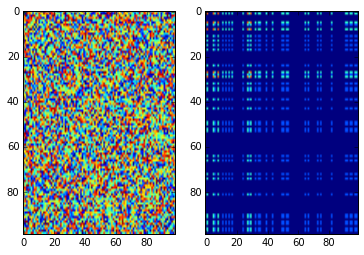In :
#strains in the yeast cross are homozygous, which is encoded [0,1] - [ref/ref,alt/alt]
#for heterozygous systems, we need to set minor=2 as the encoding is [0,1,2] - [ref/ref,ref/alt,alt/alt]
afBYxRM = calc_AF(MBYxRM,minor=1)

In :
##cut
af1000G = calc_AF(M1000G,minor=2)
afatwell = calc_AF(Matwell,minor=1)

In :
pl.figure(figsize=[15,5])

plt.subplot(1,3,1)
plot=pl.hist(afBYxRM['af'],50,normed=True)
pl.ylabel('density')
pl.title('Yeast cross')
pl.xlabel('allele frequency')

##cut
plt.subplot(1,3,2)
plot=pl.hist(af1000G['af'],50,normed=True)
pl.ylabel('density')
pl.title('1000 genomes')
pl.xlabel('allele frequency')

plt.subplot(1,3,3)
plot=pl.hist(afatwell['af'],50,normed=True)
pl.ylabel('density')
pl.title('A. thaliana')
pl.xlabel('allele frequency')

Out:
<matplotlib.text.Text at 0x12a73f410>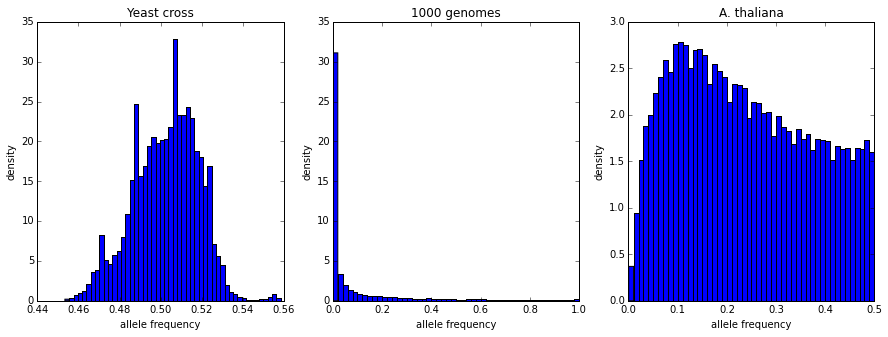## Filtering by allele frequency¶

Allele frequency is an important filter for QTL mapping. We here exlore the implications of alleele frequency filters on the number of variants available for analysis.

In :
pl.figure(figsize=[18,5])
maf = 10.**(-sp.arange(6))

plt.subplot(1,3,1)

N   = [(afBYxRM['af']>=m).sum() for m in maf]
pl.plot(maf,N,'k-')
pl.xscale('log')
pl.xlabel('maf cutoff')
pl.ylabel('number of variants')
pl.title('Yeast cross')

##cut
plt.subplot(1,3,2)

N   = [(af1000G['af']>=m).sum() for m in maf]
pl.plot(maf,N,'k-')
pl.xscale('log')
pl.xlabel('maf cutoff')
pl.ylabel('number of variants')
pl.title('1000 genomes')

plt.subplot(1,3,3)

N   = [(afatwell['af']>=m).sum() for m in maf]
pl.plot(maf,N,'k-')
pl.xscale('log')
pl.xlabel('maf cutoff')
pl.ylabel('number of variants')
pl.title('A. thaliana')

Out:
<matplotlib.text.Text at 0x12ad73290>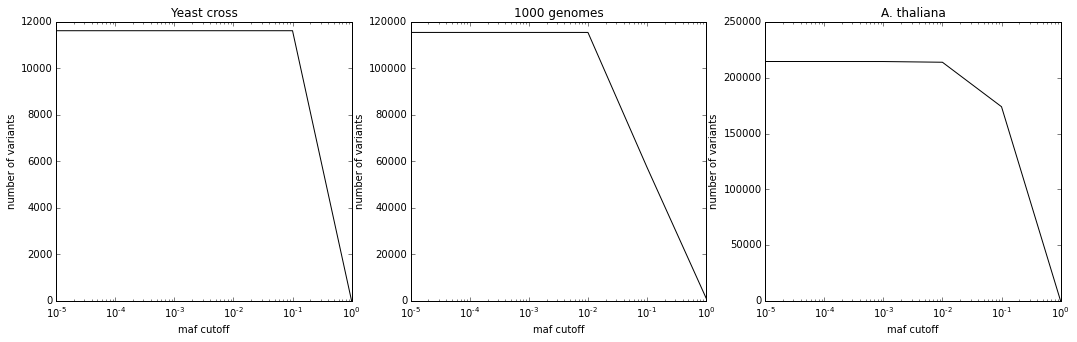In [ ]:



The resolution of genotype to phenotype mapping is affect by linkage disequilibrium. We here explore the correlation between nearby genetic markers as a function of distance.

• How does the minor allele frequency affect LD?
• How to global and local correlations compare and are related to differences in the genetic designs ?
• Is there long-range LD and if yes, what might be causes for this effect ?
In :
#Local LD plots

pl.figure(figsize=[15,5])

plt.subplot(1,3,1)
LDByxRM = calc_LD(MBYxRM,posBYxRM.values)
pl.plot(LDByxRM,LDByxRM)
pl.ylabel('r^2')
pl.xlabel('dist (bp)')
pl.title('Yeast cross')

#the calc_LD function has an optional parameter i_start, determining from how many positions the sampling started.
#you can use this to improve the resolution. try i_start=sp.arange(10) or i_start=sp.arange(100)

## CUT
maf = 0.01
plt.subplot(1,3,2)
Iaf = af1000G['af']>=maf
LDp = calc_LD(M1000G[:,Iaf],pos1000G.values[Iaf],i_start=sp.arange(100))
pl.plot(LDp,LDp)
pl.ylabel('r^2')
pl.xlabel('dist (bp)')
pl.title('1000 genomes')

plt.subplot(1,3,3)
Iaf = afatwell['af']>=maf
LDp = calc_LD(Matwell[:,Iaf],posatwell.values[Iaf],i_start=sp.arange(10))
pl.plot(LDp,LDp)
pl.ylabel('r^2')
pl.xlabel('dist (bp)')
pl.title('A. thaliana')

Out:
<matplotlib.text.Text at 0x12ae790d0>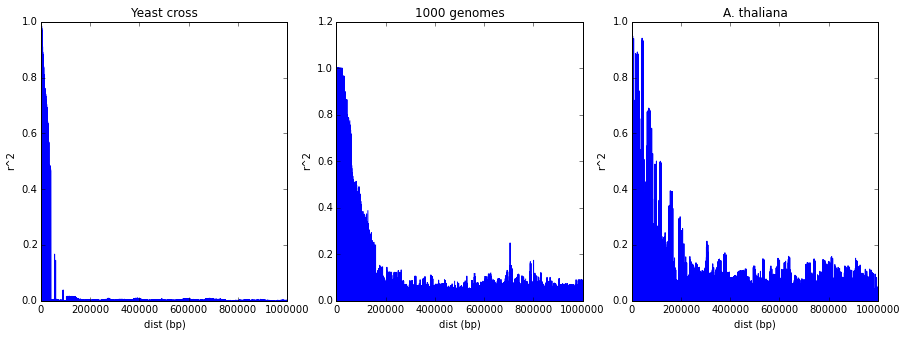## Population structure¶

Principal component analysis can be used to reveal particular relationship between samples. We here apply PCA to multiple different datasets to explore the extent of population structure.

• Apply PCA to the yeast cross, the Arabidopsis data and 1000 genomes data.
• Do you see clusters witin the data?
• If yes, what do these clusters corresond to ?
In :
# PCA

import limix.deprecated.stats.pca as pca

pl.figure(figsize=[18,5])

plt.subplot(1,3,1)
[snpsX,snpsW] = pca.PCA(MBYxRM[:,0:5000],components=10)
pl.plot(snpsX[:,0],snpsX[:,1],'k.')
pl.xlabel('PC1')
pl.ylabel('PC2')
pl.title('Yeast cross')

## cut
plt.subplot(1,3,2)
[snpsX,snpsW] = pca.PCA(M1000G[:,0:5000],components=10)
pl.plot(snpsX[:,0],snpsX[:,1],'k.')
ICEU = (population_1000G=='CEU')
IYRI = (population_1000G=='YRI')

pl.plot(snpsX[:,0][ICEU],snpsX[:,1][ICEU],'k.',label='CEU')
pl.plot(snpsX[:,0][IYRI],snpsX[:,1][IYRI],'r.',label='YRI')
pl.xlabel('PC1')
pl.ylabel('PC2')
pl.legend()
pl.title('1000 genomes')

plt.subplot(1,3,3)
[snpsX,snpsW] = pca.PCA(Matwell[:,0:5000],components=10)
pl.plot(snpsX[:,0],snpsX[:,1],'k.')
pl.xlabel('PC1')
pl.ylabel('PC2')
pl.title('A. thaliana')

Out:
<matplotlib.text.Text at 0x12b656190>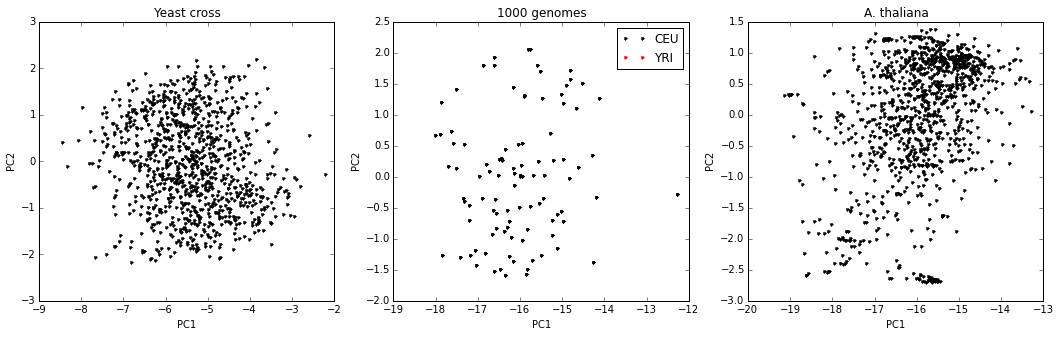In :


In :


In :


In :


In :


In :